# Triangle and Quadrilateral Worksheets

#### How to Classify Triangles and Quadrilaterals

When it comes to geometry, there are two types of shapes that we have to deal with. These include two-dimensional (2D) and three-dimensional (3D). Both 2D and 3D shapes are sub-divided into several other shapes. Circle, ovals, triangles, and quadrilaterals are all forms of 2D shapes. Among them, triangles and quadrilaterals are further categorized into several types. Triangles are polygons made using three lines. This shape has three sides and three angles. These are classified into equilateral triangles, where all three sides and all three angles are the same, isosceles triangle, where two sides and two angles are same, scalene is where none of three sides or angles are the same; and right-angle triangle, where one angle is equal to 90°. The scalene is also divided into acute-angled triangles where all three angles are less than 90° and obtuse-angled triangle, where one angle is greater than 90°. Quadrilaterals are polygons that are made of four sides. There are four types of quadrilaterals. These include squares, where all four sides are the same, and all four angles are 90°; rectangles, where two opposite sides are equal, and all four angles are 90°; parallelogram, where opposite sides are parallel and equal, and the kite is a quadrilateral where diagonals intersect at 90°.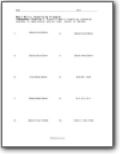###### Classifying Triangles Based on Measures

You will be given the dimensions of the figures and asked to classify each triangle.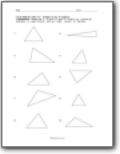###### Classifying Drawings of Triangles

Classify each triangle as isosceles, scalene, or equilateral and as right, acute, or obtuse.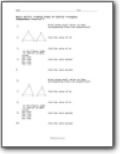###### Finding Sides of Similar Triangles

Write three equal ratios to show corresponding sides are proportional.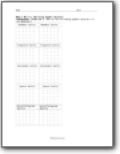###### Building Quadrilaterals

Build the following quadrilaterals in the geoboard.###### Tangram Used

You are given a shape and asked to build it using pieces. How many tangrams will you use?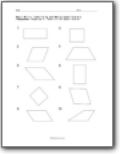###### Identifying and Making Quadrilaterals

You will be presented with a shape, identify it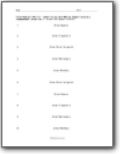###### Making Quadrilaterals

We name a shape and you draw it right there.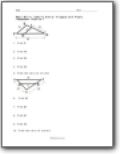###### Identify Similar Triangles with Proofs

You will given a triangle with subcuts within it. Find the missing sides.